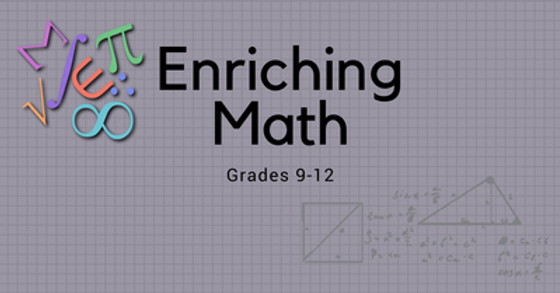top of page# MATH CLASSES# MATH CLASSES

Students develop and strengthen essential transferable skills by going through math courses.
Students should reflect on their core skills in the three areas with guidance from their parents.

• Communcation:     Communication and Collaboration

• Thinking:                 Critical, Reflective, and Creative Thinking

• Personal & Social : Personal awareness & Responsibility

Positive personal & Cultural Identity
Social awareness & ResponsibilityDevelop mathematical skills through reasoning and analyzing. Build reasoning and logic to apply multiple strategies to solve problems in both abstract and contextualized situations.

• Squares and cubes

• Cube roots

• Percents

• Rates & Ratio

• Opearations with Fractions

• Discrete linear relations

• Expressions

• Two-step equations

• Surface area & volume

• Pythagorean theorem

• Theoretical probability

• Fianancial literacy

Demonstrate and improve mathematical skills through reasoning and communicating/justifying mathematical ideas and decisions.

• Operations with rational numbers

• Exponents and exponent laws

• Operations with polynomials

• Two-variable lionear relations

• Multi-step one-variable linear equations

• Statistics in society

• Fiancial literacy## Foundations of Mathematics and Pre-Calculus 10

Accelerate mathematical skills and deductive reasoning. Investigate the relationships between quantities in various real-world contexts with skills in the following areas:

• Measurement Systems, Surface Area, and Volume

• Polynomials

• Relations and Functions

• Linear Functions

• Solving Linear Systems

• Trigonometry

## Pre-Calculus 11

Extend the understanding of relations and use more advanced algebra to solve problems through techniques such as quadratic relations and trigonometry:

• Rational Expressions

• Trigonometry

• Systems of Equations

• Sequence and SeriesPre-Calculus 12

Extend the study of functions to the connections between families of functions and learn to model the transformations of shapes of functions in various contexts.

• Transformations

• Polynomials

• Logarithms

• Trigonometric Functions

• Trigonometric Equations

• Combinatorics

AP Calculus

AP Calculus AB is equivalent to a first semester college calculus course devoted to topics in differential and integral calculus. The AP course covers topics in these areas, including concepts and skills of limits, derivatives, definite integrals, and the Fundamental Theorem of Calculus.

AP Calculus BC is equivalent to both first and second semester college calculus courses and extends the above concepts to different types of equations and introduces sequences and series.## MATH CONTEST PREPARATION

• Our experienced teachers assist students in preparing for CEMC,COMC,AMC,CCC to achieve the best results

• Encourage students to explore and discover about mathematics and computer science

• Our students participated in internationally recognized competitions

• Make your university application stand out

• Qualified competitors receive scholarship towards future studies in degree programs​

CEMC

COMC

AMC

## INSPIRING MATH (GR. 5 - GR. 8)

This program is designed to challenge students to enrich their fundamental mathematics skills. Students will put their math skills to the test by completing challenging problems and concepts, while working as a team to solve complex assignments and projects.## ENRICHING MATH (GR. 9 - GR. 12)

This program is designed to challenge students with pre-existing strong mathematical skills. Through hands-on, fun-filled activities, students will put their math skills to the test with challenging problems and concepts for preparing American and Canadian Math Competition.bottom of page